NEET  >  R.C. Mukherjee Test: States of Matter

# R.C. Mukherjee Test: States of Matter - NEET

Test Description

## 30 Questions MCQ Test Chemistry Class 11 - R.C. Mukherjee Test: States of Matter

R.C. Mukherjee Test: States of Matter for NEET 2023 is part of Chemistry Class 11 preparation. The R.C. Mukherjee Test: States of Matter questions and answers have been prepared according to the NEET exam syllabus.The R.C. Mukherjee Test: States of Matter MCQs are made for NEET 2023 Exam. Find important definitions, questions, notes, meanings, examples, exercises, MCQs and online tests for R.C. Mukherjee Test: States of Matter below.
Solutions of R.C. Mukherjee Test: States of Matter questions in English are available as part of our Chemistry Class 11 for NEET & R.C. Mukherjee Test: States of Matter solutions in Hindi for Chemistry Class 11 course. Download more important topics, notes, lectures and mock test series for NEET Exam by signing up for free. Attempt R.C. Mukherjee Test: States of Matter | 30 questions in 60 minutes | Mock test for NEET preparation | Free important questions MCQ to study Chemistry Class 11 for NEET Exam | Download free PDF with solutions
 1 Crore+ students have signed up on EduRev. Have you?
R.C. Mukherjee Test: States of Matter - Question 1

### At constant temperature, in a given mass of an ideal gas -

Detailed Solution for R.C. Mukherjee Test: States of Matter - Question 1

n,T → const
PV = const

R.C. Mukherjee Test: States of Matter - Question 2

### Three flasks of equal volumes contain CH4, CO2 and Cl2 gases respectively. They will contain equal number of molecules if -

Detailed Solution for R.C. Mukherjee Test: States of Matter - Question 2

n → constant
v → fixed
∴ P & T→ const

R.C. Mukherjee Test: States of Matter - Question 3

### A certain mass of a gas occupies a volume of 2 litres at STP. Keeping the pressure constant at what temperature would the gas occupy a volume of 4 litres -

Detailed Solution for R.C. Mukherjee Test: States of Matter - Question 3

V = 2 litre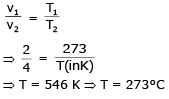R.C. Mukherjee Test: States of Matter - Question 4

If 500 ml of a gas 'A' at 1000 torr and 1000 ml of gas B at 800 torr are placed in a 2L container, the final pressure will be-

Detailed Solution for R.C. Mukherjee Test: States of Matter - Question 4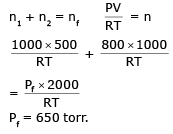R.C. Mukherjee Test: States of Matter - Question 5

Two flasks A and B of 500 ml each are respectively filled with O2 and SO2 at 300 K and 1 atm. pressure. The flasks will contain-

Detailed Solution for R.C. Mukherjee Test: States of Matter - Question 5

n = const.
no of molecules = const
same number of molecules

R.C. Mukherjee Test: States of Matter - Question 6

In the gas equation PV = nRT, the value of universal gas constant would depend only on -

Detailed Solution for R.C. Mukherjee Test: States of Matter - Question 6

R is depend upon unit of measurement

R.C. Mukherjee Test: States of Matter - Question 7

A 0.5 dm3 flask contains gas 'A' and 1 dm3 flask contains gas 'B' at the same temperature. If density of A = 3.0 gm dm-3 and that of B = 1.5 gm dm-3 and the molar mass of A = 1/2 of B, then the ratio of pressure exerted by gases is-

Detailed Solution for R.C. Mukherjee Test: States of Matter - Question 7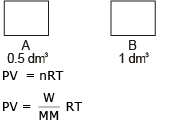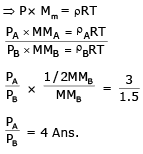R.C. Mukherjee Test: States of Matter - Question 8

When the pressure of 5L of N2 is doubled and its temperature is raised from 300K to 600K, the final volume of the gas would be-

Detailed Solution for R.C. Mukherjee Test: States of Matter - Question 8

PV = nRT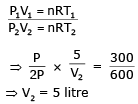R.C. Mukherjee Test: States of Matter - Question 9

If the pressure of a gas contained in a closed vessel in increased by 0.4% when heated by 1°C its initial temperature must be :

Detailed Solution for R.C. Mukherjee Test: States of Matter - Question 9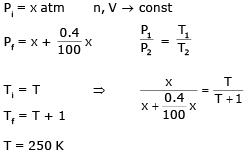R.C. Mukherjee Test: States of Matter - Question 10

A thin ballon filled with air at 47°C has a volume of 3 litre. If on placing it in a cooled room its volume becomes 2.7 litre, the temperature of room is :

Detailed Solution for R.C. Mukherjee Test: States of Matter - Question 10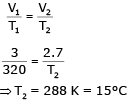R.C. Mukherjee Test: States of Matter - Question 11

If a mixture containing 3 moles of hydrogen and 1 mole of nitrogen is converted completely into ammonia, the ratio of initial and final volume under the same temperature and pressure would be:

Detailed Solution for R.C. Mukherjee Test: States of Matter - Question 11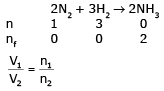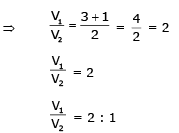R.C. Mukherjee Test: States of Matter - Question 12

SO2 at STP contained in a flask was repleced by O2 under identical conditions of pressure, temperature and volume. Then the weight of O2 will be ______ SO2.

Detailed Solution for R.C. Mukherjee Test: States of Matter - Question 12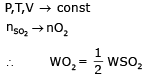R.C. Mukherjee Test: States of Matter - Question 13

Assuming that O2 molecule is spherical in shape with radius 2Å, the percentage of the volume of O2 molecules to the total volume of gas at S.T.P. is :

Detailed Solution for R.C. Mukherjee Test: States of Matter - Question 13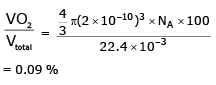R.C. Mukherjee Test: States of Matter - Question 14

Two flasks of equal volume are connected by a narrow tube (of negligible volume) all at 27°C and contain 0.70 mole of H2 at 0.5 atm. One of the flask is then immersed into a bath kept at 127°C, while the other remains at 27°C. The final pressure in each flask is :

Detailed Solution for R.C. Mukherjee Test: States of Matter - Question 14

∴ n1 +n2  = 0.7 moles    (1)
P,V = const.
n1T1 = n2T2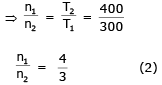n1 = 0.4 & n2 = 0.3
Icontainer PV = n1RT1 (3)
IIcontainer PV = n2RT2 (4)
using (3) & (4)
Final pressure  = 0.5714 atm

R.C. Mukherjee Test: States of Matter - Question 15

Two flasks of equal volume are connected by a narrow tube (of negligible volume) all at 27°C and contain 0.70 moles of H2 at 0.5 atm. One of the flask is then immersed into a bath kept at 127° C, while the other remains at 27°C . The number of moles of H2 in flask 1 and flask 2 are:

Detailed Solution for R.C. Mukherjee Test: States of Matter - Question 15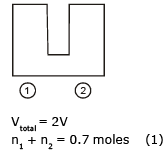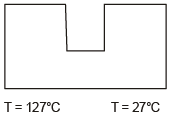P,V  = const
n1T1 = n2T2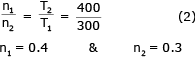R.C. Mukherjee Test: States of Matter - Question 16

A gas is heated from 0°C to 100°C at 0.1 atm pressure .If the initial volume of the gas is 10.0 l, its final volume would be :

Detailed Solution for R.C. Mukherjee Test: States of Matter - Question 16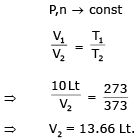R.C. Mukherjee Test: States of Matter - Question 17

Under what conditions will a pure sample of an ideal gas not only exhibit a pressure of 1 atm but also a concentration of 1 mol litre-1. [R = 0.0892 litre atm mol-1 K-1]

Detailed Solution for R.C. Mukherjee Test: States of Matter - Question 17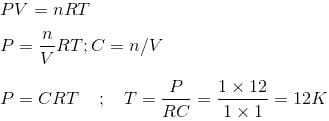R.C. Mukherjee Test: States of Matter - Question 18

A and B are two identical vessels. A contains 15 g ethane at 1atm and 298K. The vessel B contains 75 g of a gas X2 at same temperature and pressure. The vapour density of X2 is :

Detailed Solution for R.C. Mukherjee Test: States of Matter - Question 18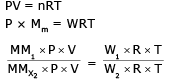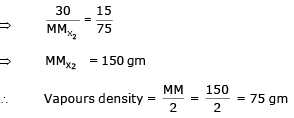R.C. Mukherjee Test: States of Matter - Question 19

The density of neon will be highest at :

Detailed Solution for R.C. Mukherjee Test: States of Matter - Question 19

PV = nRT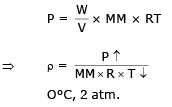R.C. Mukherjee Test: States of Matter - Question 20

A 0.5 dm3 flask contains gas A and 1 dm3 flask contains gas B at the same temperature. If density of A = 3 g/dm3 and that of B = 1.5 g/dmand the molar mass of A = 1/2 of B, the ratio of pressure exerted by gases is :

Detailed Solution for R.C. Mukherjee Test: States of Matter - Question 20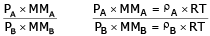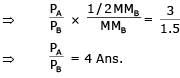R.C. Mukherjee Test: States of Matter - Question 21

A cylinder is filled with a gaseous mixture containing equal masses of CO and N2. The partial pressure ratio is:

Detailed Solution for R.C. Mukherjee Test: States of Matter - Question 21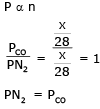R.C. Mukherjee Test: States of Matter - Question 22

A gas X diffuses three times faster than another gas Y the ratio of their densities i.e., Dx : Dy is-

Detailed Solution for R.C. Mukherjee Test: States of Matter - Question 22

Here we use Graham's law of diffusion.

Formula :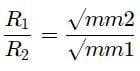Let the rate of gas Y be r1​, then the rate of gas X will be 3r1

Let the density of X and Y be Dx​ and Dy​ respectively then substituting in the formula we have: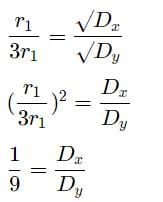The ratio is thus 1:9

R.C. Mukherjee Test: States of Matter - Question 23

At STP, a container has 1 mole of Ar, 2 moles of CO2, 3 moles of O2 and 4 moles of N2. Without changing the total pressure if one mole of O2 is removed, the partial pressure of O2 :

Detailed Solution for R.C. Mukherjee Test: States of Matter - Question 23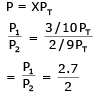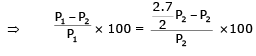⇒ 26 %
Partial pressure of O2 is changed by about 26%

R.C. Mukherjee Test: States of Matter - Question 24

Equal weights of ethane & hydrogen are mixed in an empty container at 25°C, the fraction of the total pressure exerted by hydrogen is :

Detailed Solution for R.C. Mukherjee Test: States of Matter - Question 24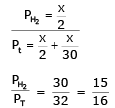R.C. Mukherjee Test: States of Matter - Question 25

A mixture of hydrogen and oxygen at one bar pressure contains 20% by weight of hydrogen. Partical presure of hydrogen will be :

Detailed Solution for R.C. Mukherjee Test: States of Matter - Question 25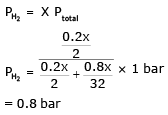R.C. Mukherjee Test: States of Matter - Question 26

A compound exists in the gaseous phase both as monomer (A) and dimer (A2). The atomic mass of A is 48 and molecular mass of A2 is 96. ln an experiment 96 g of the compound was confined in a vessel of volume 33.6 litre and heated to 237°C. The pressure developed if the compound exists as dimer to the extent of 50% by weight under these conditions will be :

Detailed Solution for R.C. Mukherjee Test: States of Matter - Question 26

WA = 48 gm
WA2 → 48 gm
PV = nRT
P ×  33.6 = (nA + nA2) RT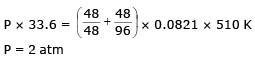R.C. Mukherjee Test: States of Matter - Question 27

Three footballs are respectively filled with nitrogen, hydrogen and helium. If the leaking of the gas occurs with time from the filling hole, then the ratio of the rate of leaking of gases (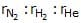) from three footballs (in equal time interval) is :

Detailed Solution for R.C. Mukherjee Test: States of Matter - Question 27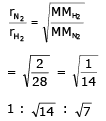R.C. Mukherjee Test: States of Matter - Question 28

The rates of diffusion of SO3, CO2, PCl3 and SO2 are in the following order-

Detailed Solution for R.C. Mukherjee Test: States of Matter - Question 28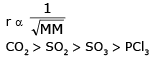R.C. Mukherjee Test: States of Matter - Question 29

20 ℓ of SO2, diffuses through a porous partition in 60 seconds. Volume of O2 diffuse under similar conditions in 30 seconds will be :

Detailed Solution for R.C. Mukherjee Test: States of Matter - Question 29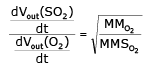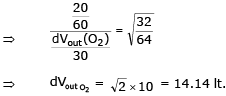R.C. Mukherjee Test: States of Matter - Question 30

See the figure :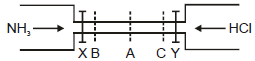The valves of X and Y opened simultaneously. The white fumes of NH4Cl will first form at :

Detailed Solution for R.C. Mukherjee Test: States of Matter - Question 30

According to Graham law of diffusion, rate is inversely proportional to root of molar mass.
Molar massNH3 =17 and Molar massHCl =36.5.
Molar Mass of HCl is more than NH3,  so it rate /speed is less.
So NH4Cl is formed at C because HCl cover less distance and NH3 travel faster and reach at C.

## Chemistry Class 11

199 videos|339 docs|229 tests
Information about R.C. Mukherjee Test: States of Matter Page
In this test you can find the Exam questions for R.C. Mukherjee Test: States of Matter solved & explained in the simplest way possible. Besides giving Questions and answers for R.C. Mukherjee Test: States of Matter, EduRev gives you an ample number of Online tests for practice

## Chemistry Class 11

199 videos|339 docs|229 tests

### How to Prepare for NEET

Read our guide to prepare for NEET which is created by Toppers & the best Teachers(Scan QR code)